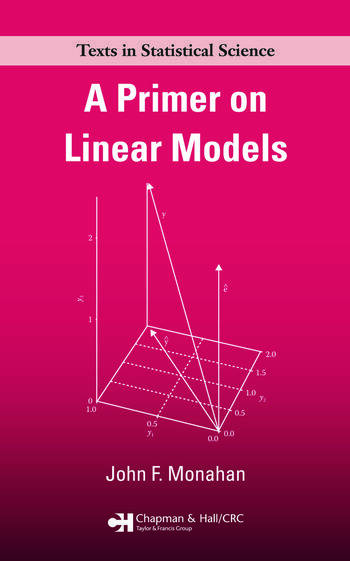# A Primer on Linear Models

## 1st Edition

John F. Monahan

Chapman and Hall/CRC
Published March 31, 2008
Textbook - 304 Pages - 6 B/W Illustrations
ISBN 9781420062014 - CAT# C6201
Series: Chapman & Hall/CRC Texts in Statistical Science

For Instructors Request Inspection Copy

was \$64.95

USD\$51.96

SAVE ~\$12.99

FREE Standard Shipping!

## Preview

### Summary

A Primer on Linear Models presents a unified, thorough, and rigorous development of the theory behind the statistical methodology of regression and analysis of variance (ANOVA). It seamlessly incorporates these concepts using non-full-rank design matrices and emphasizes the exact, finite sample theory supporting common statistical methods.

With coverage steadily progressing in complexity, the text first provides examples of the general linear model, including multiple regression models, one-way ANOVA, mixed-effects models, and time series models. It then introduces the basic algebra and geometry of the linear least squares problem, before delving into estimability and the Gauss–Markov model. After presenting the statistical tools of hypothesis tests and confidence intervals, the author analyzes mixed models, such as two-way mixed ANOVA, and the multivariate linear model. The appendices review linear algebra fundamentals and results as well as Lagrange multipliers.

This book enables complete comprehension of the material by taking a general, unifying approach to the theory, fundamentals, and exact results of linear models.

#### Instructors

We provide complimentary e-inspection copies of primary textbooks to instructors considering our books for course adoption.